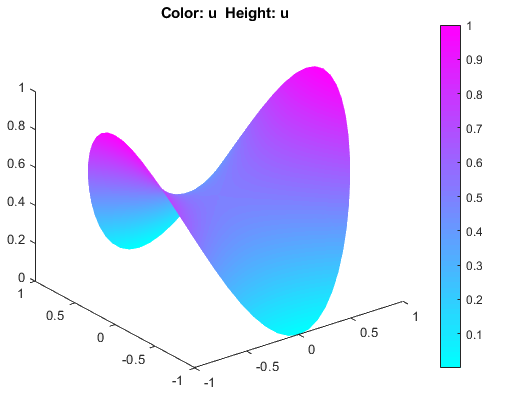Documentation

Minimal Surface Problem: PDE Modeler App

This example shows how to solve the minimal surface equation

$-\nabla \cdot \left(\frac{1}{\sqrt{1+{|\nabla u|}^{2}}}\nabla u\right)=0$

on the unit disk Ω = {(x,y) | x2 + y2 ≤ 1}, with u = x2 on the boundary ∂Ω.

This example uses the PDE Modeler app. For the programmatic workflow, see Minimal Surface Problem.

An elliptic equation in the toolbox form is

$-\nabla \cdot \left(c\nabla u\right)+au=f$

Therefore, for the minimal surface problem the coefficients are as follows:

Because the coefficient c is a function of the solution u, the minimal surface problem is a nonlinear elliptic problem.

To solve the minimal surface problem in the PDE Modeler app, follow these steps:

1. Model the surface as a unit circle.

pdecirc([0 0 1])
2. Check that the application mode is set to Generic Scalar.

3. Specify the boundary conditions. To do this:

1. Switch to boundary mode by clicking thebutton or selecting Boundary > Boundary Mode.

2. Select all boundaries by selecting Edit > Select All.

3. Select Boundary > Specify Boundary Conditions.

4. Specify the Dirichlet boundary condition u = x2. To do this, specify h = 1, r = x.^2.

4. Specify the coefficients by selecting PDE > PDE Specification or clicking the PDE button on the toolbar. Specify c = 1./sqrt(1+ux.^2+uy.^2), a = 0, and f = 0.

5. Initialize the mesh by selecting Mesh > Initialize Mesh.

6. Refine the mesh by selecting Mesh > Refine Mesh.

7. Choose the nonlinear solver. To do this, select Solve > Parameters and check Use nonlinear solver. Set the tolerance parameter to 0.001.

8. Solve the PDE by selecting Solve > Solve PDE or clicking the = button on the toolbar.

9. Plot the solution in 3-D. To do this, select PlotParameters. In the resulting dialog box, select Height (3-D plot).Partial Differential Equation Toolbox DocumentationGet trial now